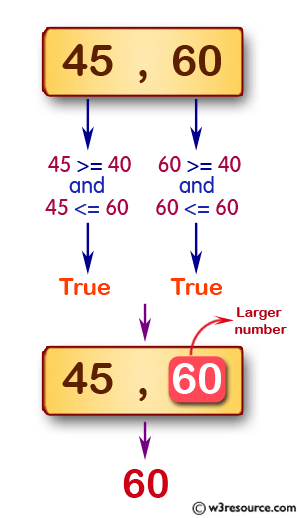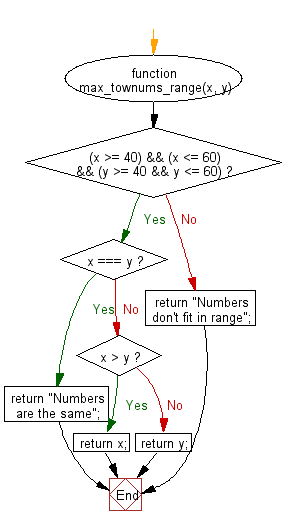# JavaScript: Find the larger number from the two given positive integers

## JavaScript Basic: Exercise-34 with Solution

Write a JavaScript program to find the larger number from the two given positive integers, the two numbers are in the range 40..60 inclusive.

Pictorial Presentation:Sample Solution:

HTML Code:

``````<!DOCTYPE html>
<html>
<meta charset="utf-8">
<meta name="viewport" content="width=device-width">
<title>JavaScript program to find the larger number from the two given positive integers, the two numbers  are in the range 40..60 inclusive.</title>
<body>

</body>
</html>
```
```

JavaScript Code:

``````function max_townums_range(x, y){
if( (x >= 40) && (x <= 60) && (y >= 40 && y <= 60) ){
if(x === y){
return "Numbers are the same";
}else if (x > y){
return x;
}else{
return y;
}
}else{
return "Numbers don't fit in range";
}
}

console.log(max_townums_range(45, 60));
console.log(max_townums_range(25, 60));
console.log(max_townums_range(45, 80));
```
```

Output :

```60
Numbers don't fit in range
Numbers don't fit in range
```

Flowchart:ES6 Version:

``````function max_townums_range(x, y) {
if (x >= 40 && x <= 60 && y >= 40 && y <= 60) {
if (x === y) {
return "Numbers are the same";
} else if (x > y) {
return x;
} else {
return y;
}
} else {
return "Numbers don't fit in range";
}
}

console.log(max_townums_range(45, 60));
console.log(max_townums_range(25, 60));
console.log(max_townums_range(45, 80));
``````

Live Demo:

See the Pen javascript-basic-exercise-34 by w3resource (@w3resource) on CodePen.

Improve this sample solution and post your code through Disqus

What is the difficulty level of this exercise?

Test your Programming skills with w3resource's quiz.

﻿

## JavaScript: Tips of the Day

Converts a string to title case

Example:

```const toTitleCase = str =>
str
.match(/[A-Z]{2,}(?=[A-Z][a-z]+[0-9]*|\b)|[A-Z]?[a-z]+[0-9]*|[A-Z]|[0-9]+/g)
.map(x => x.charAt(0).toUpperCase() + x.slice(1))
.join(' ');
console.log(toTitleCase('some_database_field_name')); // 'Some Database Field Name'
console.log(toTitleCase('Some label that needs to be title-cased')); // 'Some Label That Needs To Be Title Cased'
console.log(toTitleCase('some-package-name')); // 'Some Package Name'
console.log(toTitleCase('some-mixed_string with spaces_underscores-and-hyphens')); // 'Some Mixed String With Spaces Underscores And Hyphens'
```

Output:

```"Some Database Field Name"
"Some Label That Needs To Be Title Cased"
"Some Package Name"
"Some Mixed String With Spaces Underscores And Hyphens"
```